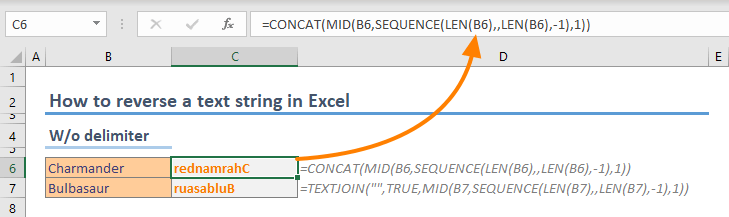In this guide, we're going to show you how to reverse a text string in Excel.

Please note that as of we are writing this article, functions like TEXTJOIN and CONCAT are available for Excel 2019 and Microsoft 365 subscribers only.

## Syntax

### CONCAT version

=CONCAT(MID(B4,SEQUENCE(LEN(<text>),,LEN(<text>),-1),1))

### TEXTJOIN version

=TEXTJOIN("",TRUE,MID(<text>,SEQUENCE(LEN(<text>),,LEN(<text>),-1),1))

## How it works

Both CONCAT and TEXTJOIN function can merge strings in arrays which was impossible with outdated CONCATENATE function back then. The difference between each function is TEXTJOIN’s ability to add a delimiter between characters. Prefer either of them based on your needs.

CONCAT(text1, [text2], …)

TEXTJOIN(delimiter, ignore_empty, text1, [text2], …)

The idea is to supply the characters into a text-merge function in reverse order. This can be done by using an array of descending numbers up to 1 (e.g., 5,4,3,2,1), using the MID function. The MID function can parse a string from a text value. All you need is to provide the index number of the character to point where you want to start parsing (start_num) and the length of characters you want to parse (num_chars).

MID(text, start_num, num_chars)

Thus, the start_num argument needs to include the descending array. The SEQUENCE function can generate a sequence of numbers as an array. It needs dimensions of the return array (row, column), start number (start_num) and the increment of each step between values (step).

=SEQUENCE(rows,[columns],[start],[step])

The last function you will need here is the LEN function which simply returns the number of characters in a given string. You need to know the number of characters to determine the number the descending sequence starts.

LEN(text)

## Examples

### Reversing a text string without delimiters

In the following example:

1. The LEN returns the number of characters for the string “Charizard” = 10
2. The SEQUENCE returns an array of descending numbers starting from 10 = {10;9;8;7;6;5;4;3;2;1}
3. The MID function return 1 character for each number in the array = {"r";"e";"d";"n";"a";"m";"r";"a";"h";"C"}
4. Finally, either the CONCAT or TEXTJOIN merges the characters in reverse order = rednamrahC

=CONCAT(MID(B6,SEQUENCE(LEN(B6),,LEN(B6),-1),1))

=TEXTJOIN("",TRUE,MID(B7,SEQUENCE(LEN(B7),,LEN(B7),-1),1))### Reversing a text string with delimiters

If you want to put a delimiter character(s) between each character of the source text, prefer using the TEXTJOIN function instead, and enter the delimiter into the first argument. For example, dots (.) separate each character in the following example.

=TEXTJOIN(".",TRUE,MID(B11,SEQUENCE(LEN(B11),,LEN(B11),-1),1))## Bonus: A custom formula to reverse a text string in Excel

The LAMBDA function allows creating custom functions using other Excel formulas. Once you add the formula as a named range and you can start using the named range as your custom function. For detailed information please check out the LAMBDA page: Function: LAMBDA

=LAMBDA(text,CONCAT(MID(text,SEQUENCE(LEN(text),,LEN(text),-1),1)))## Simplify your functions using the LET Function

Additionally, you can check out the LET function to make your functions use a single argument. You can define in-formula named ranges to replace the actual references in the formula. In the following examples, the named range text is defined in the LET function to replace cell references:

=LET(text,B20,CONCAT(MID(text,SEQUENCE(LEN(text),,LEN(text),-1),1)))

=LET(text,B21,TEXTJOIN("",TRUE,MID(text,SEQUENCE(LEN(text),,LEN(text),-1),1)))When copying this formula, only single reference needs to be changed instead of three.